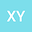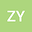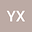Asymptotical mean-square stability of linear θ-methods for stochastic pantograph diﬀerential equations: variable stepsize and transformation approach
•••• xiaochen yang,
• Zhanwen Yang,
• Yu Xiao
xiaochen yang
Harbin Institute of Technology

Corresponding Author:19b912041@stu.hit.edu.cn

Author ProfileZhanwen Yang
Harbin Institute of Technology
Author ProfileYu Xiao
Harbin Institute of Technology
Author Profile## Abstract

The paper deals with the asymptotical mean-square stability of the linear θ-methods under variable stepsize and transformation approach for stochastic pantograph diﬀerential equations. A limiting equation for the analysis of numerical stability is introduced by Kronecker products. Under the condition which guarantee the stability of exact solutions, the optimal stability region of the linear θ-methods under variable stepsize is given by using the limiting equation, i.e., θ ∈ (1/ 2,1], which is the same to the deterministic problems. Moreover the linear θ-methods under the transformation approach are also considered and the result of the stability is improved for θ = 1 /2. Finally, numerical examples are given to illustrate the asymptotical meansquare stability under variable stepsize and transformation approach.
04 Jun 2021Published in International Journal of Computer Mathematics on pages 1-12. 10.1080/00207160.2021.1932841